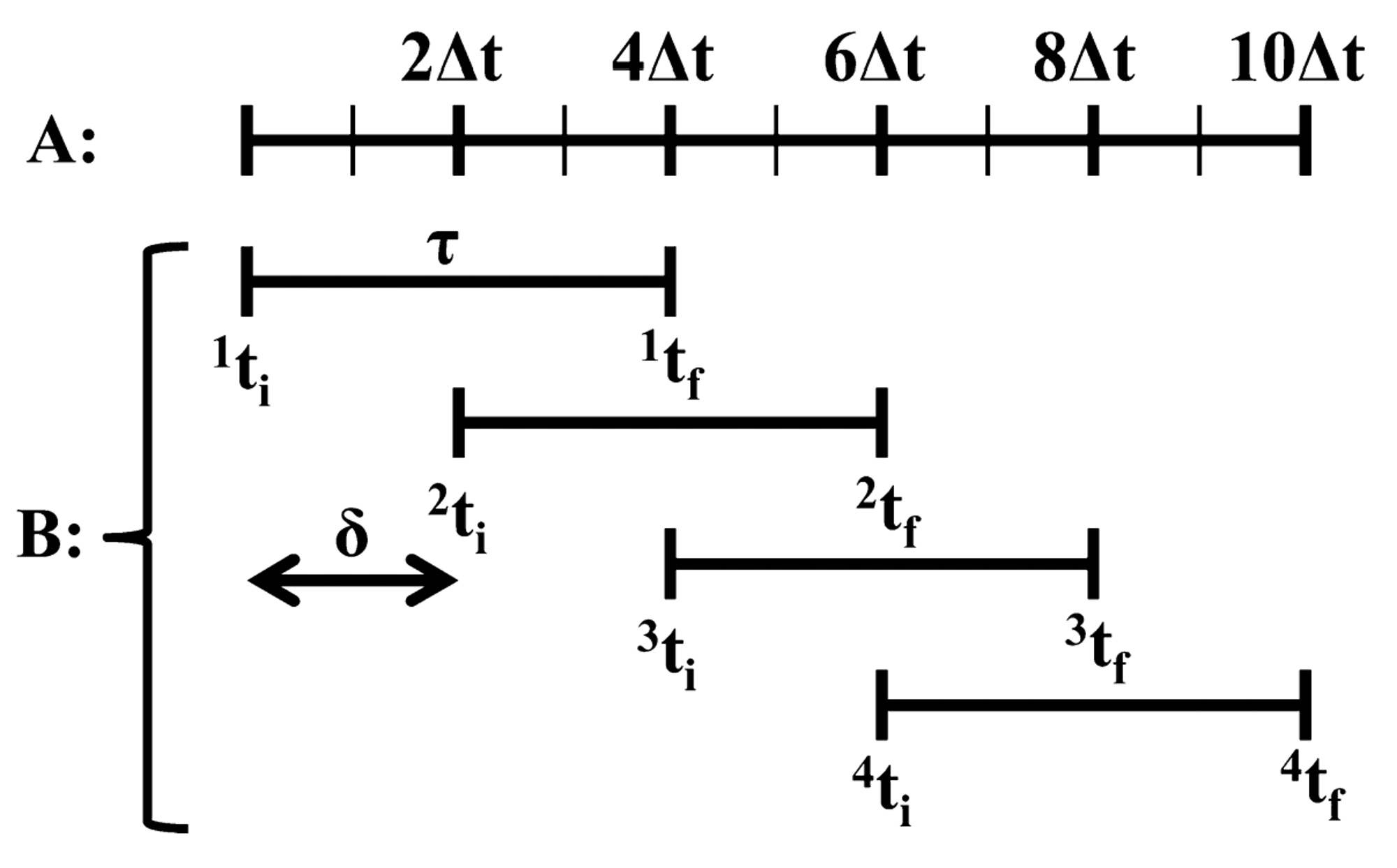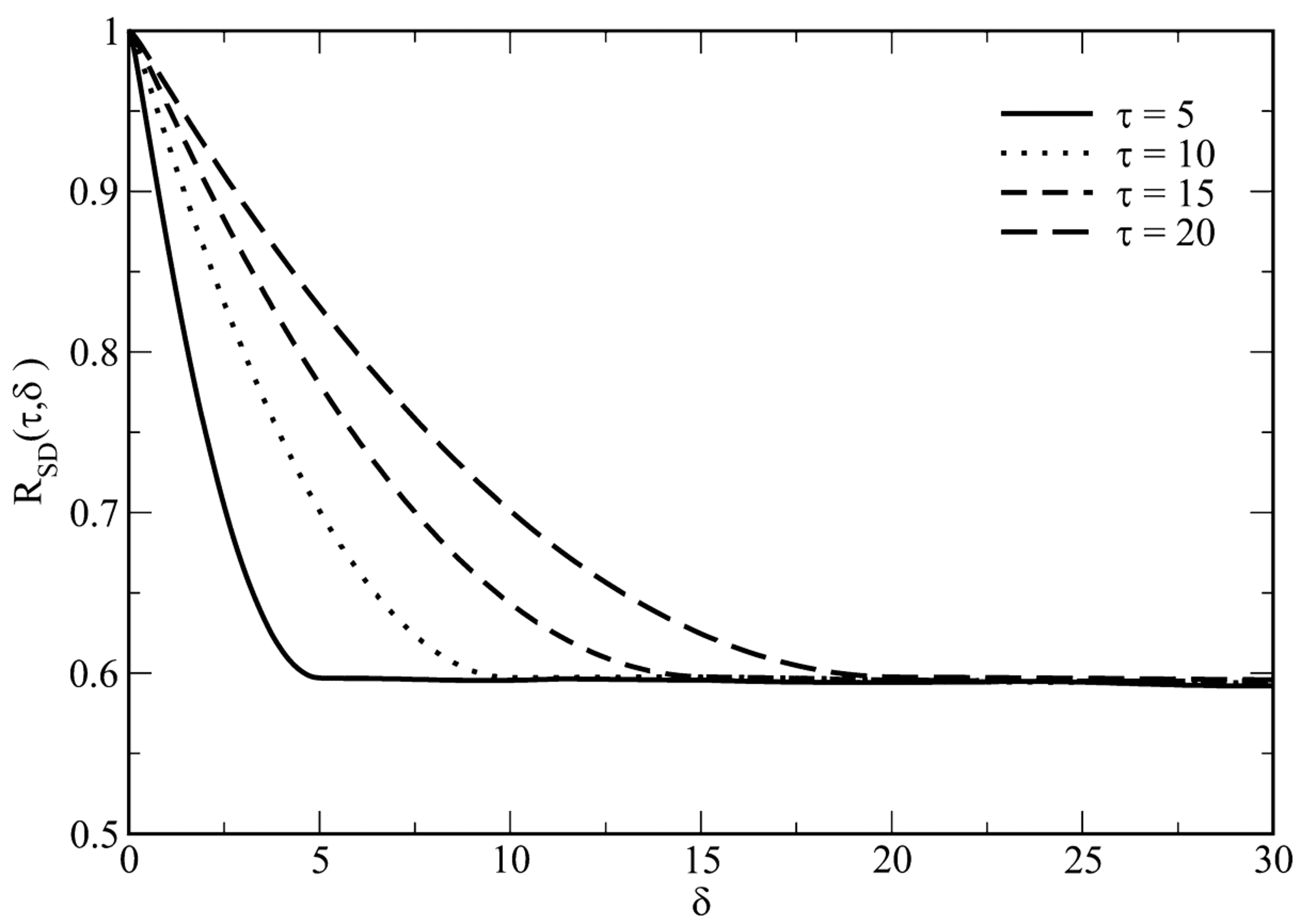墨滴2021/07/04  阅读：27  主题：默认主题

# Estimating Error in Diffusion Coefficients Derived from Molecular Dynamics Simulations

Reference paper: J. Chem. Theory Comput. 2015, 11, 4586−4592

Assumption that the simulation is long enough so that the ergodic hypothesis1 applies is not necessarily true.

If ergodic hypothesis does not stand, the molecular dynamics simulation of a Lennard-Jones fluid2 has a poor estimation on the self-diffusion coefficient.

Diffusion coeff: D of a particle undergoing random walk (self-diffusion of LJ fluid and a rigid fractal aggregate diffusion in LJ fluid) is given by Einstein's relation:

where N is the dimension of the system, is the particle position at time , refers to time origin, and the angle brackets denote the average over the time period. is the mean square displacement (MSD), which grows linearly with a sufficiently large values of .

The calculation of MSD of particles from the molecular dynamics trajectory is shown in Figure 1. If the displacement of the particles over a time interval of width is calculated such that the distance between the time origins of two consecutive time intervals is δ, then is given by:

where is the number of time intervals of width over which the average of squared displacement ( ) is calculate, is the position of particle at time .Figure 1. Schematic describing the calculation of mean squared displacement MSD(τ) over a given time interval (τ). (A) The MD trajectory where the particle coordinates are recorded at a time interval of Δt, as indicated by vertical tick marks. (B) The time intervals jtf − jti (= τ) over which the displacement of particles is calculated. Mean squared displacement is obtained by taking the mean of the square of these displacements. The separation, δ, between the time origins of two consecutive intervals is given by j+1ti − jti.

Sampling squared displacements: Independent sampling of SD is required for the determination of the diffusion coefficient of randomly diffusing particles in LJ fluid from their mean squared displacement. An appropriate value of has to be determined for ensuring the independent sampling of SD. A normalized autocorrelation function of SD was defined as a function of and given by the following equation.

Figure 2 shows that decays to a constant value as . This shows that should be sampled such that in order to ensure independent sampling of SD in the system studied.Figure 2. Normalized autocorrelation function of squared displacement samples, RSD(τ,δ) as a function of δ (time units) for different values of τ (time units). Data obtained from a MD simulation of 125 particles (NP) comprising a LJ fluid. The plot shows that autocorrelation function decays to a constant value as δ → τ. Therefore, independent sampling of squared displacements would be ensured by choosing δ ≥ τ.

1 In physics and thermodynamics, the ergodic hypothesis says that, over long periods of time, the time spent by a system in some region of the phase space of microstates with the same energy is proportional to the volume of this region, i.e., that all accessible microstates are equiprobable over a long period of time. (Wikipedia)
2 Lennard-Jones fluid is a model fluid where the forces between particles are decribed by the pairwise additive and spherically symmetric Lennard-Jones potential: Despite its simplicity, the Lennard-Jones model fluid yields a realistic representation of simple fluids.2021/07/04  阅读：27  主题：默认主题